# Numpy and Pandas in Python

## Numpy and Pandas in Python

In this article, I am going to discuss Numpy and Pandas in Python with Examples. Please read our previous article where we discussed Data Structures in Python. At the end of this article, you will understand the following pointers.

1. Learning NumPy
2. Machine Learning application
3. Introduction to Pandas
4. Creating Data Frames
5. Grouping and Sorting
6. Plotting Data
7. Converting Different Formats
8. Combining Data from Various Formats
9. Slicing/Dicing Operations.
##### Learning Numpy

NumPy is one of Python’s most essential libraries, and it’s also one of the most helpful. NumPy is capable of handling big datasets with ease. I can almost see your eyes glinting with excitement at the possibility of mastering NumPy. As data scientists or aspirant data scientists, we must have a strong understanding of NumPy and how it works in Python.

NumPy stands for Numerical Python, and it’s one of Python’s most helpful scientific libraries. It supports massive multidimensional array objects as well as a variety of tools for working with them. Other libraries, including Pandas, Matplotlib, and Scikit-learn, are built on top of this incredible library.

A collection of elements/values with one or more dimensions is known as an array. A Vector is a one-dimensional array, while a Matrix is a two-dimensional array.

N-dimensional arrays are NumPy arrays that store elements of the same type and size. It is well-known for its great performance, and as arrays grow in size, it delivers efficient storage and data operations.

pip install numpy

Now you need to import the library:

import numpy as np

np is the de facto abbreviation for NumPy used by the data science community.

A Python object is really a pointer to a memory address where all of the object’s details, such as bytes and value, are stored. Although this additional information is what makes Python a dynamically typed language, it comes with a price, which becomes apparent when keeping a big collection of objects, such as in an array.

Python lists are just an array of pointers, each pointing to a location containing the element’s information. This adds a significant amount of memory and calculation overhead. When all of the objects in the list are of the same type, most of this information is rendered superfluous!

To get around this, we utilize NumPy arrays with only homogeneous elements, that is, items of the same data type. This improves the array’s storage and manipulation efficiency. When the array has a big number of elements, such as thousands or millions, this difference becomes obvious. You can also execute element-wise operations with NumPy arrays, which is not feasible with Python lists!

When doing mathematical operations on a big amount of data, NumPy arrays are recommended over Python lists for this reason.

##### Creating a Numpy Array

Given the complexity of the problems they tackle, NumPy arrays are remarkably simple to generate. The np.array() technique is used to generate a very simple ndarray. All you have to do is pass the array’s values as a list:

np.array([1,2,3,4])

Output: array([1, 2, 3, 4])

##### NumPy arrays can be multi-dimensional too.

np.array([[1,2,3,4],[5,6,7,8]])
array([[1, 2, 3, 4],[5, 6, 7, 8]])
Here, we created a 2-dimensional array of values.

Note: A matrix is just a rectangular array of numbers with the shape N x M, where N is the number of rows in the matrix and M is the number of columns. A 2 x 4 matrix is the one you just saw.

##### Array of zeroes

The np.zeros() method in NumPy allows you to build an array of all zeros. All you have to do is pass the required array’s shape:

np.zeros(5)

Output – array([0., 0., 0., 0., 0.])

The one above is a 1-D array while the one below is a 2-D array:

```np.zeros((2,3))
array([[0., 0., 0.],
[0., 0., 0.]])
```

NumPy can be used to create an array of 1s as well by using np.ones() function.

np.ones(5, dtype=np.int32)

Output – array([1, 1, 1, 1, 11])

##### Random numbers in ndarrays

Another very commonly used method to create ndarrays is np.random.rand() method. It creates an array of a given shape with random values from [0,1):

```# random
np.random.rand(2,2)```
###### Output –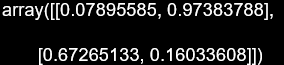##### Evenly spaced ndarray

You can use np.arange() function to create an evenly spaced array of numbers

```# create an array of odd numbers between 1 to 10
np.arange(1, 10, 2)
```

Output – array([1, 3, 5, 7, 9])

In case you want to create an array of n linearly spaced elements within a range, you can use np.linspace() function

```# create an array of 8 numbers between 0 to 2
np.linspace(0, 2, 8)
```
##### Shape of Array

The shape of a NumPy array denotes the number of rows and columns of that array respectively. You can check the shape of the array by using .shape. Here, shape will return you the number of rows, and shape will return the number of columns.

```a = np.zeros((4,2))
print(a.shape)
print("Rows :", a.shape)
print("Columns:", a.shape)
```
###### Output –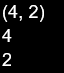##### Reshaping an Array

We can change the shape of an array without altering the data present inside an array, by using np.reshape() function.

```a = np.zeros((4,2))
np.reshape(a, (2,4))
```
###### Output –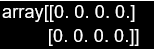##### Flattening an Array

We can convert a NumPy array of any other dimension into a single-dimensional array by using.flatten() method.

```a = np.zeros((4,2))
b = a.flatten()
```

Output – [0. 0. 0. 0. 0. 0. 0. 0.]

##### Slicing NumPy Arrays

Slicing is the process of retrieving elements from one index and moving them to another. All we have to do is transmit the index’s beginning and ending points like this: [start: end].

You can, however, take it a step further by increasing the step size. What exactly is it? So, if you wanted to publish every other element in the array, you’d set your step size to 2, which means you’d retrieve the element 2 places further from the current index. All of this would be combined into a single index, which would look like this: [start:end:step-size].

```a = np.array([9,8,7,6,5,4,3,2,1])
print(a[1:5:2])
```

Output – [8 6]

##### Sorting an Array
```a = np.array([5,6,7,4,9,2,3,7])
print(np.sort(a))
```

Output – [2 3 4 5 6 7 7 9]

##### Machine Learning application

Some of the most common applications of Machine Learning are listed below –

###### 1. Recommendation Engines –

The most popular examples of recommendation engines are – Netflix, YouTube, Spotify. Do you need a new show to watch to replace the gap left by your binge-watching? One can be found on Netflix. Check your homepage to see whether it has already happened. Netflix taps the viewing history and habits of its millions of customers to anticipate what individual viewers would likely appreciate using machine learning to curate its massive collection of TV episodes and movies.

###### 2. Self-driving cars –

These are self-driving autos that do not require a human pilot. Machine learning is used by these cars to see their surroundings, make sense of them, and forecast how others will act. With so many moving parts on the road, a sophisticated machine learning system is essential. The most common examples are – Waymo and Tesla

###### 3. Health Predicting System –

KenSci assisting caregivers are absolutely the best examples of this use case. KenSci assists caregivers with predicting which patients will become unwell so that they can intervene earlier, potentially saving money and lives. It achieves so by analyzing databases of patient information, including electronic medical records, financial data, and claims, using machine learning.

###### 4. Credit Card Worthiness Predictors –

Traditional credit card firms use a person’s FICO score and credit history to assess eligibility. However, for those with no credit history, this can be an issue. As a result, deserve — which is aimed at students and new credit card applicants — uses a machine-learning algorithm to determine credit worthiness, considering other aspects such as current financial health and behaviors.

###### 5. Farming using Computer Vision –

Blue River’s “See & Spray” technology identifies plants in farmers’ fields using computer vision and machine learning. This is very beneficial for weed detection among acres of crops. The See & Spray rig can also target specific plants and spray them with herbicide or fertilizer, as its name implies. It’s significantly more efficient and environmentally friendly than spraying a full field.

###### 6. Fashion Future Prediction –

Machine learning is used by many fashion e-commerce sites to assist buyers to find the proper size items and brands, as well as to acquire useful information about their customers. Have you ever placed an internet order for something that was far too large or small? Fit Analytics takes a customer’s body measurements and applies machine learning to recommend the best-fitting outfits. Machine learning analyses data points on the backend to provide clothes retailers with insights into everything from popular styles to typical consumer dimensions.

#### Introduction to Pandas

Pandas is an open-source library designed to make it simple and natural to work with relational or labeled data. It includes a number of data structures and methods for working with numerical data and time series. This library is based on the NumPy Python library. Pandas is quick and has a high level of performance and productivity for its users.

##### Why is it required?

Pandas are commonly employed in data science, but do you know why? This is due to the fact that pandas is used in conjunction with other data science libraries. Pandas is based on the NumPy library, which means that many NumPy structures are used or copied in Pandas. Pandas data is frequently used as input for Matplotlib plotting routines, SciPy statistical analysis, and Scikit-learn machine learning algorithms.

Pandas can be executed from any text editor, although it is advised that you use Jupyter Notebook for this because Jupyter allows you to execute code in a specific cell rather than the entire file. Jupyter also has a simple interface.

##### Benefits –
1. Data manipulation and analysis are quick and easy.
2. It is possible to load data from various file objects.
3. Missing data is easily handled.
4. Columns in DataFrame and higher-dimensional objects can be added and removed.
5. Merging and combining data sets.
6. Data sets can be reshaped and pivoted in a variety of ways.

You must import the library after the pandas have been installed on the system. You can import Pandas this way –

import pandas as pd

The Pandas are referred to as pd in this case. However, using the alias to import the library is not required; it only aids in writing less code each time a function or property is invoked.

Pandas provide two data structures for data manipulation – Series and DataFrame.

###### Series –

Pandas Series is a one-dimensional labeled array that may hold any form of data (integer, string, float, python objects, etc.). The axis labels are referred to as indexes collectively. Pandas Series is merely a column in an excel spreadsheet.

###### DataFrame –

Pandas DataFrame is a possibly heterogeneous two-dimensional size-mutable tabular data format with labeled axes (rows and columns). A data frame is a two-dimensional data structure in which data is organized in rows and columns in a tabular format. The data, rows, and columns are the three main components of a Pandas DataFrame.

##### Creating Data Frames

A Pandas DataFrame is a two-dimensional labeled data structure with columns that can be of various sorts. It is the pandas object that is utilized the most. Pandas DataFrame can be made in a variety of ways. Let’s go over each method for creating a DataFrame one by one.

###### Method1: Using lists of lists to create a Pandas DataFrame.
```import pandas as pd
# initialize list of lists
data = [['tom', 10], ['nick', 15], ['juli', 14]]
# Create the pandas DataFrame
df = pd.DataFrame(data, columns = ['Name', 'Age'])
df
```
###### Output –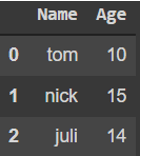##### Method2: Generating a DataFrame from a narray/lists dict

To make a DataFrame out of a dict of narrays/lists, each narray must be the same length. If the index is specified, the length index must match the length of the arrays. If no index is specified, the index is set to range(n), where n is the array length.

```import pandas as pd
# initialise data of lists.
data = {'Name':['Tom', 'nick', 'krish', 'jack'],
'Age':[20, 21, 19, 18]}
# Create DataFrame
df = pd.DataFrame(data)
df
```
###### Output –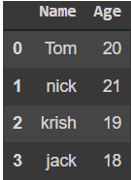##### Method 3: Arrays are used to create an index DataFrame
```import pandas as pd
# initialise data of lists.
data = {'Name':['Tom', 'Jack', 'nick', 'juli'],
'marks':[99, 98, 95, 90]}
# Creates pandas DataFrame.
df = pd.DataFrame(data, index =['rank1',
'rank2',
'rank3',
'rank4'])
df
```
###### Output –##### Method4: Using the zip() function to create a DataFrame

The list(zip()) function can be used to combine two lists. Now, call the pd.DataFrame() function to construct a pandas DataFrame.

```import pandas as pd
# List1
Name = ['tom', 'krish', 'nick', 'juli']
# List2
Age = [25, 30, 26, 22]
# and merge the two lists by using zip().
list_of_tuples = list(zip(Name, Age))
# Converting lists of tuples into pandas Dataframe
df = pd.DataFrame(list_of_tuples,
columns = ['Name', 'Age'])
df
```
###### Output –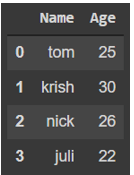#### Grouping and Sorting

##### Grouping

Pandas groupby is used to group data into categories and then apply a function to each category. It also aids in the effective aggregation of data.

The groupby() function divides data into groups depending on a set of criteria. Any of the axes can be used to separate pandas objects. Grouping is defined as a mapping of labels to group names in its most basic form.

###### Syntax –

DataFrame.groupby(by=None, axis=0, level=None, as_index=True, sort=True)

Below are some examples to demonstrate use of groupby() function. The CSV file referred in the examples can be downloaded from here.

###### Example1 –
```# importing pandas as pd
import pandas as pd

# Creating the dataframe

# Print the dataframe

# applying groupby() function to group the data on embarked value.
gk = df.groupby('Embarked')

# Let's print the first entries in all the groups formed.
gk.first()
```
###### Output –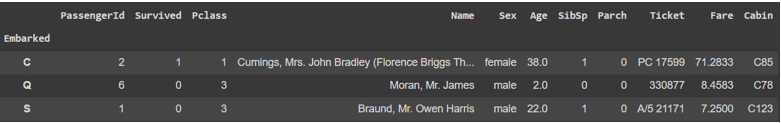##### Example2 – Forming groups on the basis of more than one category
```# First grouping based on "Embarked"
# Within each category we are grouping based on "Sex"
gkk = df.groupby(['Embarked', 'Sex'])

# Print the first value in each group
gkk.first()
```
###### Output –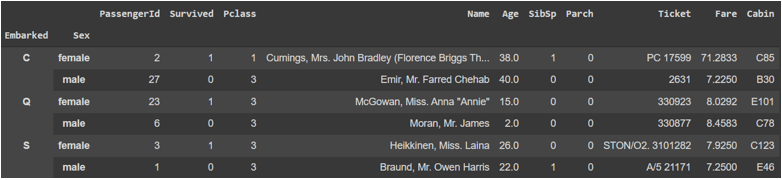##### Sorting

Pandas support the following two different types of sorting –

1. Based on index
2. Based on actual values

Let’s understand this better based on the following example of an unsorted data frame –

```import pandas as pd
import numpy as np

actual_df=pd.DataFrame(np.random.randn(10,2),index=[1,4,6,2,3,5,9,8,0,7],columns=['col2','col1'])
actual_df
```
###### Output –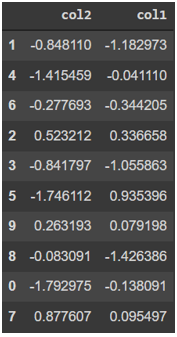##### Based on Index –

DataFrame can be sorted using the sort_index() method by giving the axis arguments and the sorting order. By default, sorting is done in ascending order on row labels.

###### Example –
```# Sort data based on index
sorted_df = actual_df.sort_index()
sorted_df
```
###### Output –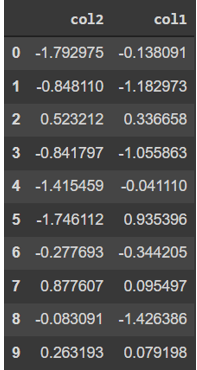##### Based on Actual Values –

sort_values(), like index sorting, is a method for sorting by values. It takes a ‘by’ argument, which specifies the column name of the DataFrame to sort the values with.

###### Example –
```# Sort data based on value
sorted_df = actual_df.sort_values(by='col1')
sorted_df
```
###### Output –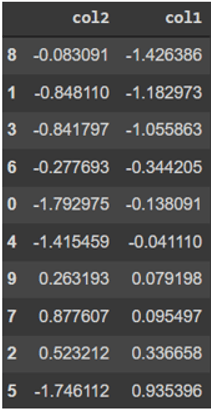##### Combining Data from Various Formats

The Series and DataFrame objects in Pandas are strong data exploration and analysis tools. A diverse strategy to merging separate datasets contributes to its power. Pandas allow you to unify and better comprehend your data as you study it by merging, joining, and concatenating datasets.

For merging DataFrames across rows or columns, use Concat().

We must indicate the axis while concatenating DataFrames. axis=0 instructs Pandas to stack the second DataFrame on top of the first. It will determine whether the column names are the same and stack them accordingly. The columns in the second DataFrame will be stacked to the RIGHT of the first DataFrame when axis=1 is used. To stack the data vertically, we must ensure that both datasets have identical columns and accompanying column formats. We want to make sure what we’re doing makes sense as we stack horizontally (i.e. the data are related in some way).

###### Example –
```titanic_df = pd.read_csv('titanic.csv')

# Stack the DataFrames on top of each other
vertical_stack = pd.concat([titanic_df, churn_df], axis=0)

# Place the DataFrames side by side
horizontal_stack = pd.concat([titanic_df, churn_df], axis=1)
```
##### Slicing and Dicing in Pandas

Using loc and iloc, we can subset the dataFrame by selecting certain rows and columns in the same command.

##### loc(label-based indexing):

We subset the dataFrame with the row and column labels using the loc technique. A list of row labels or a range of labels can be used as row labels (start bound : stop bound). And the stop bound is included in the range.

###### Example 1-

df.loc[[0,25,50],[‘Age’,’Sex’]]

###### Output –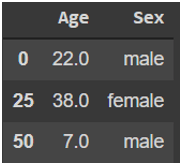df.loc[0:3,:]

###### Output –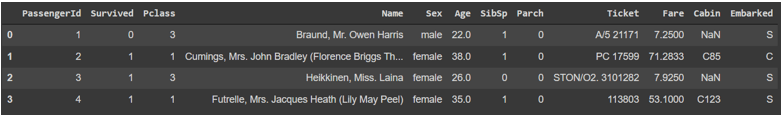##### iloc (integer-based indexing):

We will subset the data using this method based on the row and column index, which is an integer. Specifying column labels such as age, education, and so on will not work; instead, we must describe its location. The index in Python starts at 0.

df.iloc[0:3,1:6]

###### Output –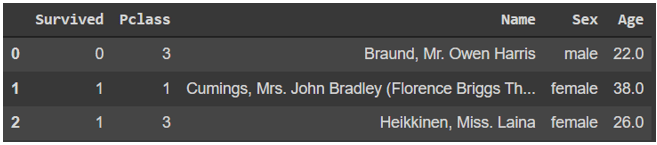In the next article, I am going to discuss Regular Expressions and Packages in Python for Data Science with Examples. Here, in this article, I try to explain Numpy and Pandas with Matplotlib and Seaborn for Data Science. I hope you enjoy this Numpy and Pandas with Matplotlib and Seaborn for Data Science article.

### 1 thought on “Numpy and Pandas in Python”

1.This article promises to learn about the interaction between pandas, matplotlib and seaborn, but it completely doesn’t focus on seaborn or matplotlib at all. This is pretty misleading.